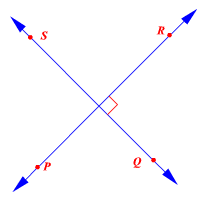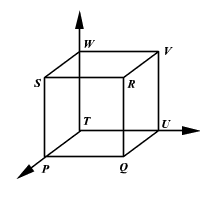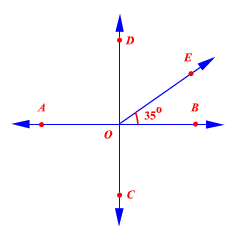# Perpendicular Lines

Perpendicular lines are lines, segments or rays that intersect to form right angles.

The symbol $\perp$ means is perpendicular to .In the figure,

$PR\perp QS$

The right angle symbol in the figure indicates that the lines are perpendicular.

In three dimensions, you can have three lines which are mutually perpendicular.The rays $\stackrel{\to }{PT},\stackrel{\to }{TU}$ and $\stackrel{\to }{TW}$ are perpendicular to each other.

Example :If $AB\perp CD$ , find $m\angle EOD$ .

Since $AB\perp CD$ , $m\angle BOD=90°$ .

By the Angle Addition Postulate,

$m\angle BOE+m\angle EOD=m\angle BOD$

Let $x°$ be the measure of $\angle EOD$ .

Then,

$35°+x°=90°$ .

Subtract $35°$ from each side.

$x°=55°$

Therefore,

$m\angle EOD=55°$ .